Disclaimer: This is an example of a student written essay.

Any opinions, findings, conclusions or recommendations expressed in this material are those of the authors and do not necessarily reflect the views of UKEssays.com.

# Calculus and Quadratic Formula to Determine Lengths

 ✅ Paper Type: Free Essay ✅ Subject: Mathematics ✅ Wordcount: 12639 words ✅ Published: 18th May 2020

INTRODUCTION

Calculus, which is one of the foremost branches of Mathematics, studies about the rates of changes. It was invented by Isaac Newton and Gottfried Leibniz in the latter half of the 17th century. It is now used in different fields and subjects such as science, economics, and engineering (Wikipedia, 2019).  For example, in Physics, calculus is used in many concepts, including motion, electricity, heat, and light. In the field of Chemistry, calculus can be used to predict reaction rates and radioactive decay. Moreover, in Biology, it is utilised to form birth and death rates. (teAchnology,2019). Calculus can also solve the problems of optimising something. Optimisation is one of the common uses of calculus in practical situations.

If you need assistance with writing your essay, our professional essay writing service is here to help!

In real life, cakes come in many different shapes and sizes. Therefore, different cakes need to be cooked in different cake tins. A cake tin manufacturer, which makes square-based or rectangular based cake tins ranging in size from “tiny” to “gigantic”, wants to optimise the volume of the cake tins. The demand for optimising the volume of the cake tins is that the bigger the volume is, the less amount of material is used so that the manufacturer can get economic benefits. To form an open top cake tin, a square from each corner of a piece of tinplate is cut, then the sides of the tinplate are folded. In this Directed Investigation, the side length of these four squares will be investigated, which value(s) of the side length would maximise the volume of the cake tins. Using various mathematical methods such as calculus and the quadratic formula, some conjectures will be made and proved. This investigation will also discuss the limitations and assumptions.

MATHEMATICAL INVESTIGATIONS & ANALYSIS

Part 1: Square Tinplate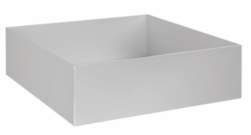After four squares from four corners of the square piece of tinplate are cut, and the sides are folded, an open top cake tin is formed.

$\mathit{x cm}$

The dimensions of the cake tin are

.

       As the length of the tinplate must be greater than 0, so $l>0$

       As the dimensions of the cake tin, which are $\mathit{x cm}$

and

, must be greater than 0, so:

So, the domains in this part are:

and

.

There are several tasks for this part:

The first task is that: it needs to be proved that when

,  the volume of the cake tin can be expressed as: $V\left(x\right)=25x–20{x}^{2}+4{x}^{3}$

When

, the width and the length of the cake tin would be

.

In this step, because

, so

is less than

$\frac{l}{2}=\frac{5}{2}=2.5$

( $0

)

The volume of a rectangular prism can be found by multiplying the length, the width and the height.

$V\left(x\right)=\mathit{lwh}$

$V\left(x\right)=\left(5–2x\right)×\left(5–2x\right)×x$

$V\left(x\right)=\left({5}^{2}–4×5x+4{x}^{2}\right)×x$

$V\left(x\right)=\left(25–20x+4{x}^{2}\right)×x$

$V\left(x\right)=25x–20{x}^{2}+4{x}^{3}$

: as required.

The second task is that calculus techniques are used to find the exact value of $x$

that will maximise the volume of the cake tin.

Firstly, the derivative function of $V\left(x\right)$

has to be found:

${V}^{‘}\left(x\right)=25–20×2x+4×3{x}^{2}=25–40x+12{x}^{2}$

Secondly, there are two ways to find the exact value of $x:$

       Because the exact value of $x$

that maximised the volume of the cake tin is required, the local maximum of $V\left(x\right)$

needs to be found. To find the local maximum of $V\left(x\right)$

, sign diagram of $V‘\left(x\right)$

is required. Therefore, the value(s) of $x$

when $V‘\left(x\right)=0$

need(s) to be found.

${V}^{‘}\left(x\right)=0⟺12{x}^{2}–40x+25=0$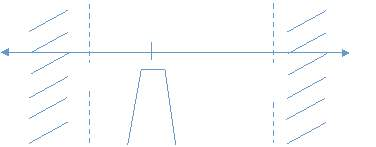Sign diagram of $V‘\left(x\right)$

:

According to the sign diagram of $V‘\left(x\right)$

, the maximum volume of the cake tin occurs when $x=\frac{5}{6}.$

Therefore, the side length of the square cuts to be made are

.

       The quadratic formula can be used to find the exact value of $x$

:

Since the derivative of $V‘\left(x\right)$

is a quadratic function, the quadratic formula can be used to solve the exact value of $x$

that optimises the volume of the cake tin.

The quadratic formula is that: if there is an equation

then:

In this case, ${V}^{‘}\left(x\right)=25–40x+12{x}^{2}$

,

As

:

$x=\frac{–\left(–40\right)±\sqrt{{\left(–40\right)}^{2}–4×12×25}}{2×12}=\frac{40±\sqrt{400}}{24}$

By doing two different ways, it is concluded that

is the exact value that needs to be found.

The third task is to repeat the process for at least two other side lengths:

a)      The first side length would be

When

, the width and the length of the cake tin would be

.

$V\left(x\right)=\mathit{lwh}$

$V\left(x\right)=\left(12–2x\right)×\left(12–2x\right)×x$

$V\left(x\right)=\left({12}^{2}–4×12x+4{x}^{2}\right)×x$

$V\left(x\right)=\left(144–48x+4{x}^{2}\right)×x$

$V\left(x\right)=144x–48{x}^{2}+4{x}^{3}$

Once again, the derivative function of $V\left(x\right)$

has to be found:

${V}^{‘}\left(x\right)=12{x}^{2}–96x+144$

The coefficients of ${V}^{‘}\left(x\right)$

are:

As

:

$x=\frac{–\left(–96\right)±\sqrt{{\left(–96\right)}^{2}–4×12×144}}{2×12}=\frac{96±\sqrt{2304}}{24}=\frac{96±48}{24}$

So,

is the exact value of $x$

that will maximise the volume of the cake tin when

.

b)      The second side length would be

(

When

, the width and the length of the cake tin would be

$V\left(x\right)=\mathit{lwh}$

$V\left(x\right)=\left(9–2x\right)×\left(9–2x\right)×x$

$V\left(x\right)=\left({9}^{2}–4×9x+4{x}^{2}\right)×x$

$V\left(x\right)=\left(81–36x+4{x}^{2}\right)×x=81x–36{x}^{2}+4{x}^{3}$

The derivative function of $V\left(x\right)$

has to be found:

${V}^{‘}\left(x\right)=12{x}^{2}–72x+81$

The coefficients of ${V}^{‘}\left(x\right)$

are:

As

:

$x=\frac{–\left(–72\right)±\sqrt{{\left(–72\right)}^{2}–4×12×81}}{2×12}=\frac{72±\sqrt{1296}}{24}=\frac{72±36}{24}$

So,

is the exact value of $x$

that will maximise the volume of the cake tin when

.

The fourth task is to present a possible conjecture based on the size of the squares to be cut from the square piece of tinplate with side lengths $\mathit{l cm}$

that will maximise the volume of the cake tin.

The following table displays the side lengths of the square tinplate

the volume of the cake tin ( $V\left(x\right)\left(c{m}^{3}\right)$

), and the side lengths of the square cuts that maximise the volume of the cake tin  $\left(\mathit{x cm}\right)$

, based on the investigation so far.

 $V\left(x\right)$ 1 5 $\frac{5}{6}\approx 0.833$ $4{x}^{3}–25{x}^{2}+20x$ 2 9 $1.5=\frac{9}{6}$ $4{x}^{3}–36{x}^{2}+81$ x 3 12 $2=\frac{12}{6}$ $4{x}^{3}–48{x}^{2}+144x$

Conjecture:Based on the calculations that have been made and recorded, the maximum value of the cake tin occurs when the value of $x$

that cuts from the tinplate is equal to one-sixth of the side length of the tinplate. The following formulas are used to present this conjecture:

The side length of the original tinplate:

The side length of the tinplate after being cut:

The volume of the cake tin: $V\left(x\right)=4{x}^{3}–4{x}^{2}l+{\mathit{xl}}^{2}$

The domain of $x$

: $0

The value of $x$

that optimise the cake tin for maximum volume:

.

The fifth task is to prove the conjecture:

Once again, calculus is used to find the derivative function of $V\left(x\right)=4{x}^{3}–4{x}^{2}l+{l}^{2}$

:

${V}^{‘}\left(x\right)=12{x}^{3}–8\mathit{xl}+{l}^{2}$

The coefficients of $V‘\left(x\right)$

are identified:

.

(As $l>0\right)$

So, by using the quadratic formula, the equation ${V}^{‘}\left(x\right)=0$

is then solved:

$x=\frac{–b±\sqrt{{b}^{2}–4\mathit{ac}}}{2a}=\frac{–\left(–8l\right)±\sqrt{{\left(–8l\right)}^{2}–4×12{l}^{2}}}{2×12}=\frac{8l±\sqrt{64{l}^{2}–48{l}^{2}}}{24}=\frac{8l±\sqrt{16{l}^{2}}}{24}$

To conclude, the maximum volume of the cake tin occurs when

.

The sixth task is to discuss the limitations and assumptions to what have been found.

In this part, there are some limitations:

1)      The maximum volume that is calculated above is actually the “outside” volume, not the “inside” volume of the cake tin. The reason is that the thickness of the original tinplate has not been considered. This can lead to the fact that the volume of the cake tin cannot be maximised.

2)      In real life, when the tinplate is cut, the sides of the cake tin may not be equal in length. There are some errors when the sides of four squares that are cut from the tinplate are measured. Therefore, the length of the sides may not be absolutely accurate.

3)      In real life, when the tinplate is folded to form the cake tin, the sides of the tinplate may not be parallel. Therefore, the cake tin may not be a rectangular prism. For that reason, the volume of the cake tin cannot be calculated using the same formula as a rectangular prism.

4)      In part A, only positive integers have been chosen to be the length of the sides of the tinplate. Surds and decimal numbers have not been considered. In real life, the side length may not be positive integers.

Part 2: Rectangular Tinplate

In part 2, a rectangular piece of tinplate where the sides are $\mathit{r cm}$

and $\mathit{s cm}$

is given. The cake tin is made by cutting a square (

from each corner, and then, the sides are folded to form the cake tin.

This part has two main tasks: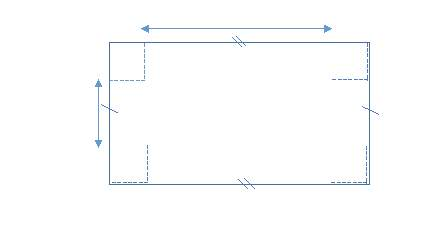The first task isto usethe ratio 1:2 for the sides of the rectangle is for investigating the relationship between $x$

and the length of each side of the rectangular tinplate so that the cake tin has a maximum volume.

Because the ratio is 1:2 for the sides of the rectangle tinplate, $s$

is assumed to be the length, and $r$

is assumed to be the width. So, $\frac{s}{r}=\frac{2}{1}\therefore s=2r$

$s$

and $r$

are the original side lengths of the tinplate, so $s$

and $r$

must be greater than 0 ( $0

).

$x$

is the side length of the squares, so $x$

must also be greater than 0 ( $x>0$

)

After being cut, the length of the tinplate is ( $s–2x$

) $\mathit{cm}$

, and the width of the tinplate is ( $r–2x$

) $\mathit{cm}$

.

Therefore, $s–2x>0$

and $r–2x>0$ $⟺x<\frac{s}{2}$

and $x<\frac{r}{2}⟺x<\frac{r}{2}$

(As $s>r$

)

So, the domains in this part are:

The dimensions of the cake tin are

Let $V\left(x\right)$

be the volume of the cake tin.

To find the relationship between $x$

and

or $x$

and $r$

, a few specific cases are considered:

1. are the dimensions of the original cake tin.

$\therefore$

The dimensions of the cake tin would be

In this case, the domain of $x$

is

$V\left(x\right)$

can be calculated by multiplying the length, the width, and the height of the cake tin.

$V\left(x\right)=\mathit{lwh}=\left(8–2x\right)\left(4–2x\right)x$

$V\left(x\right)=\left(32–8x–16x+4{x}^{2}\right)x$

$V\left(x\right)=\left(4{x}^{2}–24x+32\right)x$

$V\left(x\right)=4{x}^{3}–24{x}^{2}+32x$

To find when the maximum value of $V\left(x\right)$

occurs, calculus is used. The derivative of $V\left(x\right)$

and the sign diagram of ${V}^{‘}\left(x\right)$

are necessary.

${V}^{‘}\left(x\right)=3×4{x}^{2}–24×2x+32=12{x}^{2}–48x+32$

To find the value(s) of  $x$

that maximise(s) the volume of the cake tin, it is necessary to find when $V‘\left(x\right)=0$

.

$V‘\left(x\right)=0⇔12{x}^{2}–48x+32=0⇔3{x}^{2}–12x+8=0$

Because $V‘\left(x\right)$

is a quadratic function, the quadratic formula can be used to solve for $x$

when ${V}^{‘}\left(x\right)=0$

The quadratic formula is $x=\frac{–b±\sqrt{{b}^{2}–4\mathit{ac}}}{2a}$

.

Substitute the values of

to the quadratic formula, the value(s) of $x$

is (are) then solved:

$x=\frac{–\left(–12\right)±\sqrt{{\left(–12\right)}^{2}–4×3×8}}{2×3}=\frac{12±\sqrt{144–96}}{6}=\frac{12±\sqrt{48}}{6}=\frac{6±2\sqrt{3}}{3}$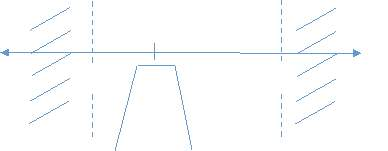Sign diagram of $V‘\left(x\right)$

:

Local maximum

According to the sign diagram, the maximum volume of the cake tin occurs when $x=\frac{6–2\sqrt{3}}{3}$

.

1. are the dimensions of the original tinplate.

$\therefore$

The dimensions of the cake tin would be

In this case, the domain of $x$

is

$V\left(x\right)=\mathit{lwh}=\left(10–2x\right)\left(5–2x\right)x$

$V\left(x\right)=\left(50–20x–10x+4{x}^{2}\right)x$

$V\left(x\right)=\left(4{x}^{2}–30x+50\right)x$

$V\left(x\right)=4{x}^{3}–30{x}^{2}+50x$

${V}^{‘}\left(x\right)=3×4{x}^{2}–30×2x+50=12{x}^{2}–60x+50$

${V}^{‘}\left(x\right)=0⇔12{x}^{2}–60x+50=0⇔6{x}^{2}–30x+25=0$

The value(s) of $x$

is(are) then solved using the quadratic formula: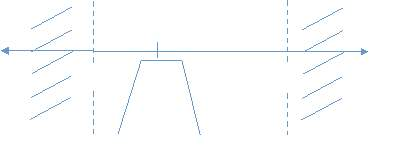Sign diagram of $V‘\left(x\right)$

:

Local maximum

According to the sign diagram, the maximum volume of the cake tin occurs when $x=\frac{15–5\sqrt{3}}{6}$

.

1. are the dimensions of the original tinplate.

$\therefore$

The dimensions of the cake tin would be

In this case, the domain of $x$

is

$V\left(x\right)=\mathit{lwh}=\left(12–2x\right)\left(6–2x\right)x$

$V\left(x\right)=\left(72–24x–12x+4{x}^{2}\right)x$

$V\left(x\right)=\left(4{x}^{2}–36x+72\right)x$

$V\left(x\right)=4{x}^{3}–36{x}^{2}+72x$

${V}^{‘}\left(x\right)=3×4{x}^{2}–36×2x+72=12{x}^{2}–72x+72$

${V}^{‘}\left(x\right)=0⇔12{x}^{2}–72x+72=0⇔{x}^{2}–6x+6=0$

The value(s) of $x$

is(are) then solved using the quadratic formula:

Local maximum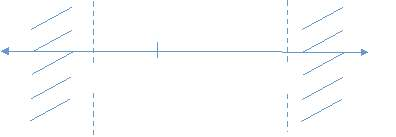Sign diagram of $V‘\left(x\right)$

: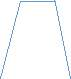According to the sign diagram, the maximum volume of the cake tin occurs when

.

The table below illustrates the values of the dimensions of the original tinplate ( $\mathit{s cm}$

and $\mathit{r cm}$

), the value of $x$

that maximise the volume of the cake tin (the data is from three cases that have been considered above), and the ratio between $x$

and r, as well as the ratio between $x$

and $s$

.

 The length of the tinplate ( $\mathit{s cm}$ ) The width of the tinplate ( $\mathit{r cm}$ ) The value of $x$ that maximise the volume of the cake tin ( $\mathit{cm}\right)$ The ratio between $x$ and $r$ ( $x:r\right)$ The ratio between $x$ and s ( $x:s\right)$ 1 $8$ $4$ $\frac{6–2\sqrt{3}}{3}$ $\frac{6–2\sqrt{3}}{3}÷4=\frac{3–\sqrt{3}}{6}$ $\frac{6–2\sqrt{3}}{3}÷8=\frac{3–\sqrt{3}}{12}$ 2 $10$ $5$ $\frac{10–5\sqrt{3}}{6}$ $\frac{15–5\sqrt{3}}{6}÷5=\frac{3–\sqrt{3}}{6}$ $\frac{15–5\sqrt{3}}{6}÷10=\frac{3–\sqrt{3}}{12}$ 3 $12$ $6$ $3–\sqrt{3}$ $\left(3–\sqrt{3}\right)÷6=\frac{3–\sqrt{3}}{6}$ $\left(3–\sqrt{3}\right)÷12=\frac{3–\sqrt{3}}{12}$

According to the table, the maximum volume of the cake tin occurs when the relationship between $x$

and $r$

is $x:r=\frac{3–\sqrt{3}}{6}\therefore x=\frac{3–\sqrt{3}}{6}×r$

, and the relationship between $x$

and $s$

is $x:s=\frac{3–\sqrt{3}}{12}\therefore x=\frac{3–\sqrt{3}}{12}×s$

.

The second taskis to summarise and to comment on any assumptions made and/or any limitations to what have been found.

Through the investigation, what has been found is that when the ratio between the width and the length of the original tinplate is $2:1$

, the ratio between $x$

and $r$

is $1:\frac{3–\sqrt{3}}{6}$

, and the ratio between $x$

and $s$

is $1:\frac{3–\sqrt{3}}{12}$

.

Together with the limitations which have been mentioned in part 1, in this part, there are some other limitations to what have been found:

1)      The ratios between $x$

and $r$

, and between $x$

and $s$

are irrational numbers. In real life, it is impossible to measure accurately that ratios, so the volume of the cake tin may not be maximised.

2)      This part only considers the ratio $2:1$

between the length and the width of the rectangular tinplate. However, in real life, the ratio could be different.

CONCLUSION

In this investigation, the relationship between the value of $x$

(the side length of the square cuts to be made) and the length of the side of the tinplate investigated. Part A investigated the case that the given tinplate is square-based, whereas part B investigated the case that the given tinplate is rectangular. Part A found out that when $x$

is equal to the value of the length of the tinplate divided by six, the volume of the open top cake tin will be maximised. Part B found out that when the ratio for the sides of the rectangular tinplate is $1:2$

, the maximum volume of the cake tin occurs when the relationship between $x$

and the width of the tinplate is $x:r=1:\frac{3–\sqrt{3}}{6}$

.

To extend this investigation, it is necessary to consider more cases. For instance, in part B, the ratio of the sides of the tinplate could be changed. Therefore, it would be suitable for real life.

For further investigation, it should be concentrated on Part B: the relationship between $x$

and $r$

when the ratio between $r$

and $s$

can be any ratios, which means a general formula between $x$

and $r$

can be investigated so that that formula would be applicable for real life, for any tinplate.

Bibliography

View all

## DMCA / Removal Request

If you are the original writer of this essay and no longer wish to have your work published on UKEssays.com then please:

Related Services

Prices from

S\$203

Approximate costs for:

• 1000 words
• 7 day deliveryHumanity University

Dedicated to your worth and value as a human being!

Related Lectures